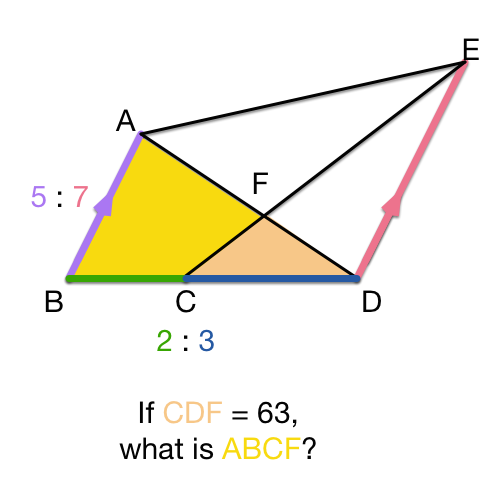Geometry Level 4In the figure, $AFD$, $BCD$ and $CFE$ are straight lines. It is given that $AB$ is parallel to $ED$, $AB : ED = 5 : 7$ and $BC : CD = 2:3$. If the area of $\triangle CDF$ is $63 \text{cm}^2$, then what is the area of $ABCF$?

×